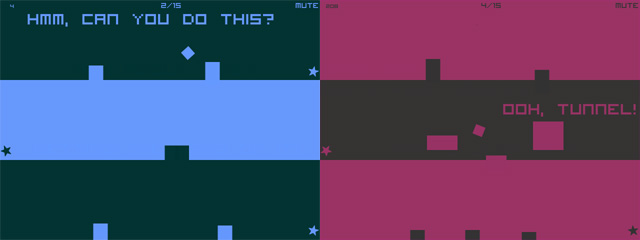# Create a Flash game like Space is Key with a lot of room for customization

Did you ever play Space is Key by Christopher Jeffrey and its sequels?It’s a quick one button game which can be used to learn some programming basics, so I am showing you this little prototype which allows a lot of customization such as player speed, jump height, jump rotation, number of floors and some minor features.

I am not using any physics engine because there’s no need in a game like this one, so let me show you the fully commented source code:

```package {
import flash.display.Sprite;
import flash.events.Event;
import flash.events.MouseEvent;
public class Main extends Sprite {
// the square!! the hero of the game
private var theSquare:TheSquare;
// square's horizontal speed in pixels/frame
private var xSpeed:Number=4;
// square's jump height, in pixels
private var jumpHeight:Number=40;
// square's jump width, in pixels. xSpeed must divide jumpWidth
private var jumpWidth:Number=120;
// rotation performed at every jump, in degrees
private var jumpRotation:Number=180;
// time passed since the player started jumping, in frames
private var jumpTime:Number=0;
// is the player jumping?
private var isJumping:Boolean;
// simple degrees to radians conversion
// array containing all various floor y positions, in pixels
private var floorY:Array=[92,184,276,368,460];
// floor I am currently moving on
private var currentFloor:Number;
// floor height, in pixels
private var floorHeight:Number=20;
// x position where the level starts, in pixels
private var levelStart:Number=0;
// x position where the level ends, in pixels
private var levelEnd:Number=640;
public function Main() {
drawBG();
init();
}
private function drawBG():void {
// simply drawing all floors, as rectangles from levelStart to levelEnd, height floorHeight
var floorCanvas:Sprite=new Sprite();
for (var i:Number=0; i<floorY.length; i++) {
floorCanvas.graphics.lineStyle(1,0x440044);
floorCanvas.graphics.beginFill(0x440044);
floorCanvas.graphics.drawRect(levelStart,floorY[i],levelEnd-levelStart,floorHeight);
floorCanvas.graphics.endFill();
}
}
private function init():void {
// we start on floor zero.
currentFloor=0;
// we aren't jumping
isJumping=false;
// adding and placing the square
theSquare=new TheSquare();
theSquare.x=levelStart;
theSquare.y=floorY[currentFloor]-theSquare.height/2;
// event listeners to update the game and jump
}
private function jump(e:MouseEvent):void {
// if we aren't jumping... then JUMP!!
if (! isJumping) {
jumpTime=0;
isJumping=true;
}
}
private function update(e:Event):void {
// temp variable to let us know if we are on an odd or even floor
var mod:Number=currentFloor%2
// updating square x position
theSquare.x+=xSpeed*(1-2*mod);
// if the square reached the end of the floor...
if (theSquare.x>levelEnd&&mod==0||theSquare.x<levelStart&&mod==1) {
// move onto next floor
currentFloor++;
// even or odd?
mod=currentFloor%2
// we start on the ground
isJumping=false;
theSquare.rotation=0;
theSquare.x=levelEnd*mod+levelStart*(1-mod);
theSquare.y=floorY[currentFloor]-theSquare.height/2;
// if we just finished the lowest floor...
if (currentFloor>floorY.length-1) {
// start the game again
removeEventListener(Event.ENTER_FRAME,update);
stage.removeEventListener(MouseEvent.CLICK,jump);
removeChild(theSquare);
init();
}
}
// if we are jumping...
if (isJumping) {
// calculating the number of frames we will be jumping
var jumpFrames:Number=jumpWidth/xSpeed;
// calculating how many degrees should the square rotate at each frame
var degreesPerFrame:Number=jumpRotation/jumpFrames*(1-2*mod);
// calculating how may radians we have to apply to sine trigonometry function to simulate player jump
// anohter frame jumping...
jumpTime++;
// updating rotation
theSquare.rotation+=degreesPerFrame;
// updating y position
// if we jumped enough...
if (jumpTime==jumpFrames) {
// ... just stop jumping
isJumping=false;
theSquare.y=floorY[currentFloor]-theSquare.height/2;
}
}
}
}
}```

As you can see, I did not use any tween or special library, so the code can be easily ported in your preferred language.

And this is the result:

Try to jump with the mouse (yeah, no space here). No obstacles at the moment, I will introduce them in next step. If you are a fan of Space is Key series, you will notice I also fixed the jumping rotation when moving from right to left.

Edit: Got a mail from Chris, he told me « Also wanted to address the left/right jump that you ‘fixed’. It was actually designed and intended that way to do doing the ‘backflip’ instead of the ‘frontflip’ on some levels throws the player off and adds to the difficulty. »

214 GAME PROTOTYPES EXPLAINED WITH SOURCE CODE
// 1+2=3
// 10000000
// 2 Cars
// 2048
// Avoider
// Ballz
// Block it
// Blockage
// Bloons
// Boids
// Bombuzal
// Breakout
// Bricks
// Columns
// CubesOut
// Dots
// DROP'd
// Dudeski
// Eskiv
// Filler
// Fling
// Globe
// HookPod
// Hundreds
// InkTd
// Iromeku
// Lumines
// Magick
// MagOrMin
// Maze
// Memdot
// Nano War
// Nodes
// o:anquan
// Ononmin
// Pacco
// Phyballs
// Platform
// Poker
// Pool
// Poux
// Pudi
// qomp
// Racing
// Renju
// SameGame
// Security
// Sling
// Slingy
// Sokoban
// Splitter
// Sproing
// Stack
// Stringy
// Sudoku
// Tetris
// Threes
// Toony
// Turn
// TwinSpin
// vvvvvv
// Wordle
// Worms
// Yanga
// Zhed
// zNumbers